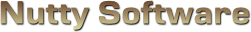Sorry, it appears you don't have support for WebGL.

In order to run this demo, you must meet the following requirements.

• You have a WebGL compatible video card with the latest drivers.
• Your video card is not blacklisted. You can check the current blacklist on Khronos.

Some browsers may require additional configuration in order to get WebGL to run. If you are having problems running this demo, visit the following sites.

Enable

#### Steps

When you render graphics in linear space, the resulting image you see is actually darker than it should be. This is because your monitor is applying a gamma to the pixels. To account for this, you must apply the inverse gamma to the pixel before sending it to the monitor. The result you see is the correct brightness.

The left side shows a gamma corrected render and the right side shows a gamma uncorrected render. The correctly rendered image is actually the one on the right side. Why? Because textures are usually already gamma corrected. The image on the left shows the texture being gamma corrected twice, causing it to be brighter than it should be.

When you use gamma corrected textures with linear lighting and gamma corrected fragments, you end up with an incorrect result. The texture is gamma corrected twice, causing the final render to appear brighter than it should be.

To render the correct result, you must uncorrect the texture by applying a gamma on it. You could do this as a pre-process to save on performance from runtime conversions. The linearized texture can then be used throughout your renderer. In your final fragment shader, you apply the inverse gamma to the pixel to produce the correct illumination.

To calibrate the perceived gamma, adjust the brightness and contrast controls such that the gradients below fall within an acceptable range. You should only just barely notice a difference between each of the black and white gradient blocks. This test works best when running in fullscreen with a completely dark background.

							
/// <summary>
/// </summary>

/// <summary>
/// Material source structure.
/// <summary>
struct MaterialSource
{
vec3 Ambient;
vec4 Diffuse;
vec3 Specular;
float Shininess;
vec2 TextureOffset;
vec2 TextureScale;
};

/// <summary>
/// Attributes.
/// <summary>
attribute vec3 Vertex;
attribute vec2 Uv;
attribute vec3 Normal;

/// <summary>
/// Uniform variables.
/// <summary>
uniform mat4 ProjectionMatrix;
uniform mat4 ViewMatrix;
uniform mat4 ModelMatrix;
uniform vec3 ModelScale;

uniform MaterialSource Material;

/// <summary>
/// Varying variables.
/// <summary>
varying vec4 vWorldVertex;
varying vec3 vWorldNormal;
varying vec2 vUv;
varying vec3 vViewVec;

/// <summary>
/// <summary>
void main ()
{
// Transform the vertex
vWorldVertex = ModelMatrix * vec4(Vertex * ModelScale, 1.0);
vec4 viewVertex = ViewMatrix * vWorldVertex;
gl_Position = ProjectionMatrix * viewVertex;

// Setup the UV coordinates
vUv = Material.TextureOffset + (Uv * Material.TextureScale);

// Rotate normal
vWorldNormal = normalize(mat3(ModelMatrix) * Normal);

// Calculate view vector (for specular lighting)
vViewVec = normalize(-viewVertex.xyz);
}


							
/// <summary>
/// Vertex shader for rendering a 2D plane on the screen. The plane should be sized
/// from -1.0 to 1.0 in the x and y axis. This shader can be shared amongst multiple
/// </summary>

/// <summary>
/// Attributes.
/// <summary>
attribute vec3 Vertex;
attribute vec2 Uv;

/// <summary>
/// Uniform variables.
/// <summary>
uniform mat4 ProjectionMatrix;
uniform mat4 ViewMatrix;
uniform mat4 ModelMatrix;
uniform vec3 ModelScale;

/// <summary>
/// Varying variables.
/// <summary>
varying vec2 vUv;

/// <summary>
/// <summary>
void main ()
{
vec4 worldVertex = ModelMatrix * vec4(Vertex * ModelScale, 1.0);
vec4 viewVertex = ViewMatrix * worldVertex;
gl_Position = ProjectionMatrix * viewVertex;

vUv = Uv;
}


							
/// <summary>
/// </summary>

#ifdef GL_ES
precision highp float;
#endif

/// <summary>
/// Light source structure.
/// <summary>
struct LightSource
{
vec3 Position;
vec3 Attenuation;
vec3 Direction;
vec3 Colour;
float OuterCutoff;
float InnerCutoff;
float Exponent;
};

/// <summary>
/// Material source structure.
/// <summary>
struct MaterialSource
{
vec3 Ambient;
vec4 Diffuse;
vec3 Specular;
float Shininess;
vec2 TextureOffset;
vec2 TextureScale;
};

/// <summary>
/// Uniform variables.
/// <summary>
uniform int NumLight;
uniform LightSource Light;
uniform MaterialSource Material;
uniform sampler2D Sample0;

/// <summary>
/// This gamma value is used for "uncorrecting" or "linearizing" a texture before
/// mixing it with the fragment.
/// <summary>
uniform float Gamma;

/// <summary>
/// Varying variables.
/// <summary>
varying vec4 vWorldVertex;
varying vec3 vWorldNormal;
varying vec2 vUv;
varying vec3 vViewVec;

/// <summary>
/// <summary>
void main ()
{
// vWorldNormal is interpolated when passed into the fragment shader.
// We need to renormalize the vector so that it stays at unit length.
vec3 normal = normalize(vWorldNormal);

vec3 colour = Material.Ambient;
for (int i = 0; i < 4; ++i)
{
if ( i >= NumLight )
break;

// Calculate diffuse term
vec3 lightVec = normalize(Light[i].Position - vWorldVertex.xyz);
float l = dot(normal, lightVec);
if ( l > 0.0 )
{
// Calculate spotlight effect
float spotlight = 1.0;
if ( (Light[i].Direction.x != 0.0) || (Light[i].Direction.y != 0.0) || (Light[i].Direction.z != 0.0) )
{
spotlight = max(-dot(lightVec, Light[i].Direction), 0.0);
float spotlightFade = clamp((Light[i].OuterCutoff - spotlight) / (Light[i].OuterCutoff - Light[i].InnerCutoff), 0.0, 1.0);
spotlight = pow(spotlight * spotlightFade, Light[i].Exponent);
}

// Calculate specular term
vec3 r = -normalize(reflect(lightVec, normal));
float s = pow(max(dot(r, vViewVec), 0.0), Material.Shininess);

// Calculate attenuation factor
float d = distance(vWorldVertex.xyz, Light[i].Position);
float a = 1.0 / (Light[i].Attenuation.x + (Light[i].Attenuation.y * d) + (Light[i].Attenuation.z * d * d));

colour += ((Material.Diffuse.xyz * l) + (Material.Specular * s)) * Light[i].Colour * a * spotlight;
}
}

// Note: Lighting is performed in linear space, but textures are nonlinear gamma corrected. As such,
// we need to 'uncorrect' or linearize the texture before applying it to the fragment. Later, in another shader,
// we will perform gamma correction on the fragment before sending it to the monitor.
//
// Optionally, you could linearize all your textures before they're loaded into WebGL, thus eliminating the need
// for uncorrecting the texture at runtime. This would improve performance and avoid data loss (gamma correction is
// lossy).
vec4 texColour = texture2D(Sample0, vUv);
texColour.xyz = pow(texColour.xyz, vec3(Gamma));

gl_FragColor = clamp(vec4(colour, Material.Diffuse.w), 0.0, 1.0) * texColour;
}


							
/// <summary>
/// Fragment shader for rendering a 2D plane on the screen.
/// </summary>

#ifdef GL_ES
precision highp float;
#endif

/// <summary>
/// Uniform variables.
/// <summary>
uniform vec2 ImageSize;
uniform vec2 TexelSize;
uniform vec4 Colour;
uniform sampler2D Sample0;

/// <summary>
/// Varying variables.
/// <summary>
varying vec2 vUv;

/// <summary>
/// <summary>
void main ()
{
gl_FragColor = texture2D(Sample0, vUv);
}


							
/// <summary>
/// Fragment shader to modify brightness, contrast, and gamma of an image.
/// </summary>

#ifdef GL_ES
precision highp float;
#endif

/// <summary>
/// Uniform variables.
/// <summary>
uniform float Brightness;	// 0 is the centre. < 0 = darken, > 1 = brighten
uniform float Contrast;		// 1 is the centre. < 1 = lower contrast, > 1 is raise contrast
uniform float GammaCutoff;	// UV cutoff before rendering the image uncorrected.
uniform float InvGamma;		// Inverse gamma correction applied to the pixel

uniform sampler2D Sample0;	// Colour texture to modify

/// <summary>
/// Varying variables.
/// <summary>
varying vec2 vUv;

/// <summary>
/// <summary>
void main ()
{
// Get the sample
vec4 colour = texture2D(Sample0, vUv);

colour.xyz = colour.xyz + Brightness;

colour.xyz = (colour.xyz - vec3(0.5)) * Contrast + vec3(0.5);

// Clamp result
colour.xyz = clamp(colour.xyz, 0.0, 1.0);

// Apply gamma, except for the alpha channel
if ( vUv.x < GammaCutoff )
colour.xyz = pow(colour.xyz, vec3(InvGamma));

// Set fragment
gl_FragColor = colour;
}



# Gamma Correction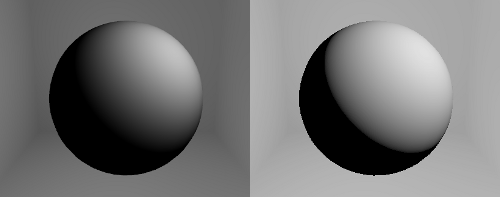Uncorrected vs corrected gamma rendering.

## Introduction

When you view an image on your monitor, the RGB pixels that make up the image are altered in such a way to better accommodate human vision. Human vision is more sensitive to shadows then it is for midtones or highlights. Hardware manufactures account for this nonlinear behaviour by applying a power curve to the pixels shown on the screen. The power value used is called the gamma value. As a result of this automatic adjustment made by your monitor, it's important for people involved in graphics and media to account for gamma correction, which produces the correct illumination. The objective of this article is to discuss what is gamma correction and how it's done.

## What is gamma?

Gamma is a power curve your monitor uses to adjust the output signal in order to better accommodate human vision.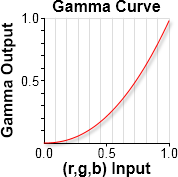This power curve represents a standard gamma of 2.2. What this curve shows you is that the gamma outputs are much darker than the RGB inputs. A pixel with an intensity value of 0.5 outputs with an intensity of approx. $0.5^{2.2}$ = 0.21, less than half as much as the input. Another way to look at this is that 50% of the colour space is dedicated to covering light intensities up to 21% and only 27% covers light intensities above 50%. We really do love the dark! To calculate the output intensities, you run the pixel inputs through a power function.

$o = i^{gamma}$

Where

$o$ is the output value.

$i$ is the input value.

$gamma$ is the gamma value used by your monitor.

Note that not all monitors are perfectly calibrated to a gamma of 2.2. Some manufacturers use different numbers such as 2.4, 2.0, 1.8, and so on. While it's ideal to ensure you use the correct values, often using the 2.2 standard can be sufficient. Ultimately, you must convince the user to calibrate their monitor. The quickest and perhaps best way to do this is to offer a monitor calibration tool in your game. It's quick and easy for the gamer to use and they don't have to worry about altering their desktop experience once they leave your game. Once you've gathered the proper gamma value, you can use this in your shaders to render the correct results.

## What is gamma correction?

Gamma is the act of your monitor altering your pixel inputs, gamma correction is the act of inverting that process for linear RGB values so that the final output remains linear. For example, if you calculated the light intensity of an object is 0.5, you don't store the result as 0.5 in the pixel. Store it as $0.5^{1.0 /2.2} = 0.73$. When you send 0.73 to the monitor, it will apply a gamma on the value and produce $0.73^{2.2}\ = 0.5$, which is what you want. To do this, you apply the inverse gamma function.

$o = i^{1.0 / gamma}$

Where

$o$ is the output value.

$i$ is the input value.

$gamma$ is the gamma value used by your monitor.

This has the following effect on the power curve.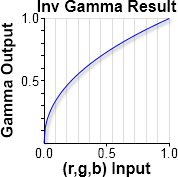=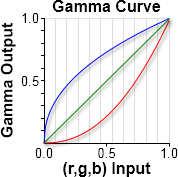The blue line represents the inverse gamma curve you need to apply to your pixels before they're sent to the monitor. When your monitor applies its gamma curve (red line) to the pixels, the result is a linear line (green line) that represents your intended RGB pixel values. When people skip this step, what ends up happening is the linear RGB values get darkened. It's not the true value that your shader computed.

The following example gradient displays shades of grey from 0 to 255.## How to implement gamma correction?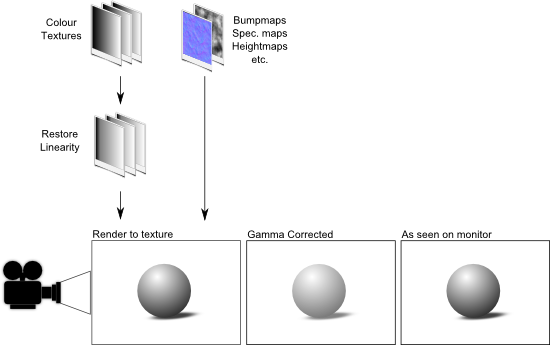The best and robust way to implement gamma correction is as a post-processing shader that takes as input a texture and calculates the inverse gamma on each pixel and stores the result in the framebuffer. This means you have to render your scene to a texture and send that to the gamma correction shader. If performance is an issue and you want to minimize the use of framebuffer objects or multiple render passes, you could intelligently put your gamma correction routine in your final output shader, such as your transform and lighting shader. This will help improve performance, but it can become inflexible if you have multiple rendering techniques and you must duplicate your gamma correction code to accommodate all the possible render paths.

You also need to "uncorrect" or "linearize" most of your colour textures. When you use editors like Gimp and Photoshop, you have to remember that what you see on the screen is the gamma adjusted pixel values. Things are darker then they actually are. When you save that image to disk, you're saving the gamma adjusted values, not the actual linear RGB values. When you import coloured textures in your game, you must "uncorrect" them to restore their linearity before using them in your shaders. You do that by applying the gamma to each pixel (not the inverse gamma value). For performance and even precision reasons (gamma correction is a lossy calculation), you should do this as a pre-process where all of your textures are uncorrected and saved to disk. Not all images have to be uncorrected, only the gamma encoded ones. Automatically generated images like normals maps do not need to be uncorrected.

## Gamma Calibration

The following test can be used to determine the monitor's gamma value. The test uses an image with black and white interlaced lines and a gamma corrected grey block in the middle. Half of the lines are black (off) and half of them are white (full power) so the average output from the monitor is 50%. 50% of 256 is 128, but 128 is a linear RGB value. We need to find the gamma corrected value to use. $0.5^{1.0 / 2.2} = 0.729 * 255 = 186$, which is the colour of the grey block in the middle. When standing back several feet, the image should appear as one colour if your monitor is calibrated to the gamma value 2.2.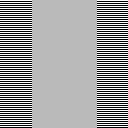Gamma 2.2 monitor test image

To test for other gamma values, you need to adjust the colour of the grey block in the middle to match with the gamma value you are testing against. This test can be difficult to perform with LCD screens since the viewing angle can alter one's perception. Care must also be taken not to stretch or filter the image in your rendered frame as this will corrupt the test.

You can't change a monitor's gamma value, but you can modify the preceived gamma by adjusting the brightness and contrast values to bring the shades of grey to within an acceptible result. The calibration involves getting the user to distinguish between shades of grey that are close together. When you place pure black (RGB = 0) and almost black (RGB = 1) side by side, you should be able to see a faint difference between the two. For some people or poor monitors, this can be a challenging task. Often separating the RGB values by 5 points should be sufficient to spot a difference.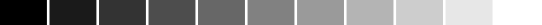This is a simple gradient. The user should be able to depict different shades throughout. If any of them are difficult to distinguish, the user will need to adjust the brightness and contrast settings to fix the result.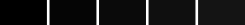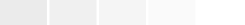Blacks and Whites Test with RGB increments of 5

Often the whites test is discarded in favour of the blacks test as it's easier on the eyes, but it's an important test to perform if you want to keep the brightness and contrast settings in check. To much or little of either can cause unnecessary over or underexposure. The objective of these tests are simple. Pure black (RGB = 0) should not be seen when mixed with a pure black background. The next black in the sequence (RGB = 5) should be slightly observable, but not too much, and so on. The user should adjust the brightness and contrast settings until all shades are comparitively noticeable. Another way to perform this test is to present the user with a screenshot from a scene in your game. The screenshot should have highs (lights) and lows (shadows) with instructions provided to the user on how to adjust their brightness and contrast settings to view the image properly.

## References

The source code for this project is made freely available for download. The ZIP package below contains both the HTML and JavaScript files to replicate this WebGL demo.

The source code utilizes the Nutty Open WebGL Framework, which is an open sourced, simplified version of the closed source Nutty WebGL Framework. It is released under a modified MIT license, so you are free to use if for personal and commercial purposes.